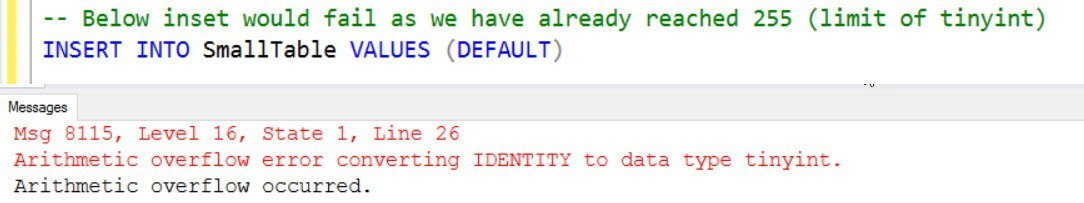# SQL SERVER – FIX : Msg 8115, Level 16, Arithmetic Overflow Error Converting IDENTITY to Data Type INT

One of my friends who is a SharePoint expert told that he is getting overflow error while doing check-in on the document. He was confident that the error is related to SQL and that’s why he contacted me. I used profiler and found the table which had the problem and fixed that as well. This blog is an outcome of that work done. Let us see the error related to Arithmetic Overflow Error.

What is an overflow? Whenever we try to insert a value in a column which is more than the limit of data type, we will get an overflow error. In my case, it was an identified column, which was defined as INT and the below are the variations of error when it comes to IDENTITY columns. It all depends on the data type of the column. The number of values permitted would be dependent on data type.

Msg 8115, Level 16, State 1, Line 25
Arithmetic overflow error converting IDENTITY to data type tinyint.
Arithmetic overflow occurred.
Msg 8115, Level 16, State 1, Line 25
Arithmetic overflow error converting IDENTITY to data type smallint.
Arithmetic overflow occurred.
Msg 8115, Level 16, State 1, Line 25
Arithmetic overflow error converting IDENTITY to data type int.
Arithmetic overflow occurred.
Msg 8115, Level 16, State 1, Line 25
Arithmetic overflow error converting IDENTITY to data type bigint.
Arithmetic overflow occurred.

Here are the steps to reproduce. Keep in mind that tinyint has limit of 255 so if we try to insert 256 in tinyint column, it would fail.

```USE [tempdb]
GO
IF OBJECT_ID('SmallTable') is NOT NULL
DROP TABLE [SmallTable]
GO
CREATE TABLE [dbo].[SmallTable] (
[i] [tinyint] IDENTITY(1, 1) PRIMARY KEY NOT NULL
,[j] [nchar](20) NULL
) ON [PRIMARY]
GO
ALTER TABLE [dbo].[SmallTable] ADD CONSTRAINT [DF_SmallTable_j] DEFAULT(N'SQLAuthority')
FOR [j]
GO
SET NOCOUNT ON
GO
-- Inserting and Deleting the rows.
INSERT INTO SmallTable VALUES (DEFAULT)
DELETE FROM SmallTable
GO 255
-- Below inset would fail as we have already reached 255 (limit of tinyint)
INSERT INTO SmallTable VALUES (DEFAULT)
/*
Msg 8115, Level 16, State 1, Line 21
Arithmetic overflow error converting IDENTITY to data type tinyint.
Arithmetic overflow occurred.
*/
-- check the rows in table and since we are inserting and deleting, its having 0 rows.
SELECT * FROM SmallTable
```

Here is the screenshot of SSMS## WORKAROUND/SOLUTION

In our above example, we have zero rows so how to verify the identity value? Refer my earlier blog.

SQL SERVER – Find Current Identity of Table

```DBCC CHECKIDENT('dbo.SmallTable')
```

Here is the output

Checking identity information: current identity value ‘255’, current column value ‘NULL’.

DBCC execution completed. If DBCC printed error messages, contact your system administrator.
Here is the command which I ran to fix the issue

```DBCC CHECKIDENT('dbo.SmallTable',reseed,0)
```

You can read more about the command over here SQL SERVER – DBCC command to RESEED Table Identity Value – Reset Table Identity

I have selected value as TINYINT as its ready to demonstrate the error. On SharePoint issue which I worked, it was integer and due to amount of project, documents and check-ins, they hit the limit.

Have you seen more variations of above error? Please share via comments.

Reference: Pinal Dave (http://blog.SQLAuthority.com)

#### Related Posts

•October 22, 2019 5:29 pm

IF I Run the following on MS SQL Management Studio using MSSQL2008:
SELECT 2147483646+1 AS [Int]
SELECT 2147483646+2 AS [BigInt]

The first is OK but the second gives this err:
Msg 8115, Level 16, State 2, Line 2
Arithmetic overflow error converting expression to data type int.

Is there a setting for BIGINT?
Another problem with same Sys:
SELECT 58*100 MultiPly, 58*10^2 MultiPlyPower
Returns
MultiPly, MultiPlyPower
5800 582

•Gabe Lawrence
November 25, 2019 9:46 pm

@Padraig SQL Server automatically sees a number as “INT”, so 2147483646 needs converted to BIGINT before doing an operation on it.

SELECT convert(bigint,2147483646)+2

•Gabe Lawrence
November 25, 2019 10:13 pm

Oops, my previous post concerning the MultiPlyPower result was wrong. After seeing the result (582), my mind saw the carrot (^) as a plus (+). I believe it’s called a “DERP” moment :-P

Look into “Bitwise Operators” (https://docs.microsoft.com/en-us/sql/t-sql/language-elements/bitwise-exclusive-or-transact-sql?view=sql-server-ver15)

The carrot (^) is Exclusive Or

Summary:
58*10 = 580 = Binary 1001000100
2 = Binary 10

10010000100
00000000010 Exclusive Or result is:
10010000110 = 582 decimal